Next: Statistics Up: Noise and statistics in Previous: Noise and statistics in

### Photon noise calculation

It is notoriously difficult to get the photon noise calculation right for polarimetry. We therefore include a reasoned example which we believe to be correct.

In the standard staring mode of measuring linear polarization, one Stokes parameter is derived from four spectra, recorded in two CCD images, for halfwave plate position angles differing by 45 degrees. Let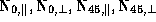be the number of photons detected per spectral resolution element in the o and e spectra for the two CCD exposures. The Stokes parameter, for example, is then given by the formula in Section 3.2.

When integration times for both exposures are the same and the level of polarization is small,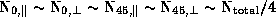, where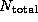is the total number of photons (per spectral resolution element) in the two exposures of the two spectra. In that case R1 and Q0.

Photon statistics gives an uncertainty of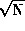for each of the N. The fractional error in each N is therefore. For multiplication and division, the fractional errors add in quadrature, hence the fractional error in Ris, the fractional error in R is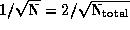. Since R1, the absolute error in R-1 is also. Since R+1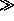R-1, the fractional error inis dominated by that in R-1, which is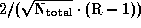. Finally, this makes the absolute error in: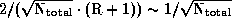.

For example, in order to determine one Stokes parameter to a degree-of-polarization accuracy of 0.005 (e.g.= 2.50.5 % or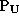= 1.00.5 % or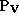= 0.10.5 %), \ 4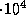photons per resolution element are required. To obtain both linear Stokes parameters with that accuracy, we need two such observations. With the present La Palma CCDs, maximum recorded output, which is close to saturation charge, is of order 6electrons per pixel. It is advisable to stay somewhat below this in single exposures, and to average over pixels and/or repeated observations as necessary for the desired accuracy.Next: Statistics Up: Noise and statistics in Previous: Noise and statistics in

manuals store
Tue Oct 7 17:34:45 BST 1997